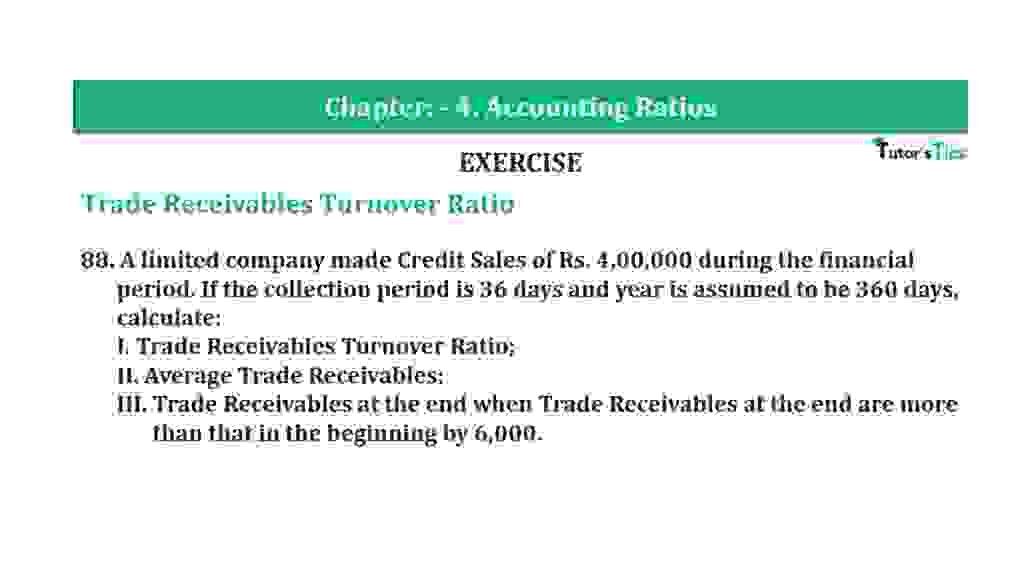# Question 88 Chapter 4 of +2-B – T.S. Grewal 12 ClassQuestion 88 Chapter 4 of +2-B

Trade Receivables Turnover Ratio

88. A limited company made Credit Sales of Rs. 4,00,000 during the financial
period. If the collection period is 36 days and year is assumed to be 360 days, calculate:
I. Trade Receivables Turnover Ratio;
II. Average Trade Receivables;
III. Trade Receivables at the end when Trade Receivables at the end are more than that in the beginning by 6,000.

### The solution of Question 88 Chapter 4 of +2-B: –

 Debt Collection Period = 360 Trade Receivables Turnover Ratio
 36 = 360 Trade Receivables Turnover Ratio = 360 36 = 10 Times

 (ii)Trade Receivables Turnover Ratio = Net Credit Sales Average Trade Receivable
 10 = Rs. 4,00,000 Average Trade Receivable = Rs. 4,00,000 10 Average Trade Receivable = Rs. 40,000

 (iii) Opening Trade Receivables = X = x + 6,000 Average Trade Receivable = Opening Trade Receivable + Closing Trade Receivable 2 Rs. 40,000 = X + (x+ Rs. 6,000) 2 Rs. 80,0000 = 2x + Rs. 6,000 2x = Rs. 80,000 – Rs. 6,000 2x = Rs. 74,000 X = Rs. 74,000 2 X = Rs. 37,000 Opening Trade Receivables = Rs. 37,000 Closing Trade Receivables = Rs. 37,000 + 6,000 = Rs. 43,000

Balance Sheet: Meaning, Format & Examples

Thanks, Please Like and share with your friends

Comment if you have any question.

Also, Check out the solved question of previous Chapters: –

### T.S. Grewal’s Double Entry Book Keeping (Vol. II: Accounting for Companies)T.S. Grewal’s Analysis of Financial Statements